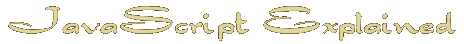Start | Time | IF | Date Object | Arrays | Avoid the work | Hide email | Random | Intigrate HTML | TestThe Math Object
Random Changes

To keep people coming back it is sometimes nice to have your index page different each time.
For instance :
On my personal pages Geoff's World I play a different midi randomly chosen each time the page is reloaded.

To do this it is a simple matter of using JavaScript 'random' to choose a number and then accessing an array with that number.

There are a couple of things that we must understand first.

JavaScript random will give you a number between 0 and 1
 choice=Math.random();

Not much good to us like that but if we multiply it by the number of choices we need it begins to make sense
 choice=Math.random()* 4;
Last thing is to make the number an integer getting rid of all that superfluous stuff after the decimal point
For this we use 'Math.round'
 choice=Math.round(Math.random()* 4);

What we have done is combine three steps in one statement which makes sense.
It could have been written
 choice = Math.random; choice = choice * 4; choice = Math.round (choice );
We now have a number between 0 and 4 ( Remember this is a computer language so you have 5 choices )
We can access an array with the answer to alter the experience on each reload.
Recapping : Here is the script


Next : Interact with HTML

Home